#### Formal calculations

The second phase is solved using formal algebraic computations. This means that the particular vector fields, differentiation, manifolds, and so on, can be ignored. The concepts involve pure algebraic manipulation. To avoid confusion with previous definitions, the term formal will be added to many coming definitions. Recall from Section 4.4.1 the formal definitions of the algebra of polynomials (e.g.,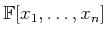). Let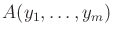denote the formal noncommutative algebra15.11 of polynomials in the variables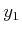,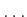,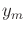. The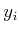here are treated as symbols and have no other assumed properties (e.g, they are not necessarily vector fields). When polynomials are multiplied in this algebra, no simplifications can be made based on commutativity. The algebra can be converted into a Lie algebra by defining a Lie bracket. For any two polynomials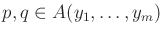, define the formal Lie bracket to be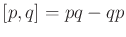. The formal Lie bracket yields an equivalence relation on the algebra; this results in a formal Lie algebra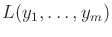(there are many equivalent expressions for the same elements of the algebra when the formal Lie bracket is applied). Nilpotent versions of the formal algebra and formal Lie algebra can be made by forcing all monomials of degree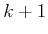to be zero. Let these be denoted by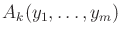and, respectively. The P. Hall basis can be applied to obtain a basis of the formal Lie algebra. Example 15.18 actually corresponds to the basis of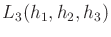using formal calculations.

Steven M LaValle 2020-08-14